PARALLELDCCIRCUITS PARALLEL DC CIRCUITS

A circuit in which two or more electrical resistances, or loads, are connected across the same voltage source is a parallel circuit. The parallel circuit differs from the series circuit in that more than one path is provided for current flow - the more paths added in parallel, the less opposition to flow of electrons from the source. In a series circuit the addition of resistance increases the opposition to current flow. The minimum requirements for a parallel circuit are the following:
 (1) a power source. (2) conductors. (3) a resistance or load for each current path. (4) two or more paths for current flow. Figure 8-62 shows a parallel circuit with three paths for current flow. Points A, B, C, and D are connected to the same conductor and are at the same electrical potential. In a similar manner, points E, F, G, and H are at the same potential. Since the applied voltage appears between points A and E, the same voltage is applied between points B and F, points C and G, and between points D and H. Thus, when resistors are connected in parallel across a voltage source, each resistor has the same applied voltage, although the currents through the resistors may differ depending on the values of resistance. The voltage in a parallel circuit may be expressed as follows: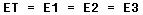Where ET is the applied voltage, E1 is the voltage across R1, E2 is the voltage across R2, and E3 is the voltage across R3 (figure 8-62).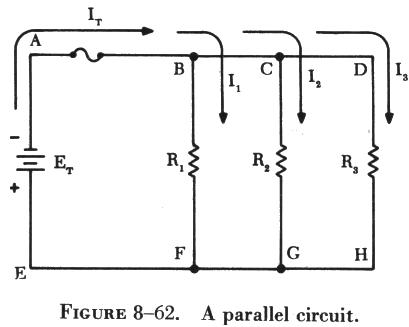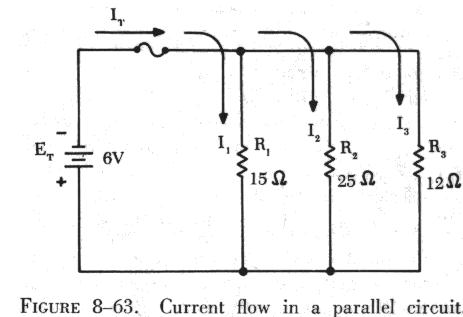The current in a parallel circuit divides among the various branches in a manner depending on the resistance of each branch (see figure 8-63). A branch containing a small value of resistance will have a greater current flow than a branch containing a high resistance. Kirchhoff's current law states that the current flowing toward a point is equal to the current flowing away from that point. Thus, the current flow in a circuit may be expressed mathematically as follows: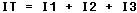where IT is the total current and I1, I2, and I3 are the currents through R1, R2, and R3, respectively. Kirchhoff's and Ohm's law can be applied to find the total current flow in the circuit shown in figure 8-63.

The current flow through the branch containing resistance R1 is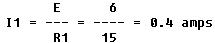The current through R2 is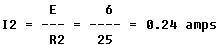The current through R3 is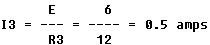The total current, IT, is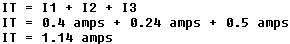In a parallel circuit, IT = I1 + I2 + I3. By Ohm's law the following relationships can be obtained: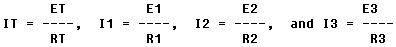Substituting these values in the equation for total current,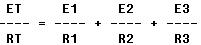In a parallel circuit ET = E1 = E2 = E3. Therefore,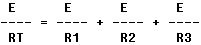Dividing through by E gives,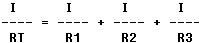This equation is the reciprocal formula for finding the total or equivalent resistance of a parallel circuit. Another form of the equation may be derived by solving for RT.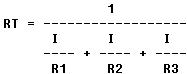An analysis of the equation for total resistance in a parallel circuit shows that RT is always less than the smallest resistance in a parallel circuit. Thus a 10 ohm, a 20 ohm, and a 40 ohm resistor connected in parallel have a total resistance of less than 10 ohms.

If there are only two resistors in a parallel circuit, the reciprocal formula is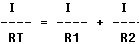Simplified, this becomes: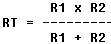This simplified, shorter formula can be used when two resistances are in parallel. Another method can be used for any number of resistors in parallel if they are of equal resistance. The resistance value of one resistor is divided by the number of resistors in parallel to determine the total resistance. Expressed mathematically this becomes: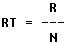Where RT is the total resistance, R is the resistance of one resistor, and N is the number of resistors.